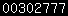24-GHz Path Loss From an article by KA1GT  (adapted for narrowband calculations by PAoEHG) Propagation at 24 GHz is different from that at lower frequencies because there is significant attenuation of signals due to absorption of energy by both oxygen and water vapour. Absorption due to oxygen rises slowly from a value of 0.004 dB/km at 1 GHz to 0.03 dB/km at 30 GHz.  Above 30 GHz, there is a sharp peak at 60 GHz, where attenuation rises to 15 dB/km. At 24 GHz, attenuation by atmospheric oxygen is approximately 0.0235 dB/km at a barometric pressure of 760-mm Hg and a temperature of 20 ° C.   Absorption due to water vapour, at 24 GHz, is considerably higher than that due to oxygen ; in fact, 24 GHz is close to a peak in the water spectrum. At a water concentration of 10 g/M³ (corresponding to 54% relative humidity at 20°C, attenuation is approximately 0. 175 dB/km.Attenuation due to water vapour is a strong function of temperature and humidity, as can be seen from Fig. 1. Over a long path of several hundred kilometres, there can be a difference of as much as 100 dB in path loss between a hot, humid day and a cold, dry day. Thus, there is an additional element involved in planning DX contacts on 24 GHz: Not only must the path be considered, but weather may also play an important part in making a contact. As an example, consider a pair of stations on 24 GHz using 100 mW narrowband and 50 cm parabolic dish antennas. What is the maximum path they can work? First, assumptions must be made about the equipment. A noise figure of 3 dB will be assumed for the receivers with an IF bandwidth of 3 kHz typical values for a      narrowband system. A 3 dB noise figure corresponds to a noise temperature of about 288° K, which, combined with a 3 kHz receiver bandwidth, leads to a receiver sensitivity of around - 169 dBW.  Assuming the parabolic antennas are 50 % efficient, they will show a gain of 40.7 dB. A 100 mW transmitter has a power output of -10 dBW.  Let's assume that a received signal-to-noise ratio of 10 dB will be required for a readable signal. It follows that the path loss a successful contact is given by   Path loss < Tp + G - Rs - SNR (Eq. 1)     Where   Tp is the transmitter power                 G is the combined antenna gains                 Rs is the receiver sensitivity                 SNR is the minimum signal-to-noise ratio required In this case, a path-loss capability of about 230 dB is indicated for the equipment discussed above. If there were no atmospheric absorption, this would give a path capability of more than 300.000 km line of sight (if such a path could be found!). Note,. that free-space path loss is given by Free Space Path Loss = 32.45 + 20 log f + 20 log d (Eq. 2)     where: f is the frequency in megahertz                                                                           d is the path length in kilometres When atmospheric absorption is taken into account, the range of the equipment is considerably reduced. Take, for example, conditions of 20°C and 50% relative humidity. From Fig. 1, it can be seen that this corresponds to about 0. 165-dB/km attenuation due to water vapour and 0.0235. dB/km due to oxygen. With a path loss capability of 230 dB, this gives a range of about 320 km. At this range, the free-space path loss is 170 dB, the loss due to water vapour attenuation is 52.8 dB, and the loss due to oxygen attenuation is 7.6 dB; giving a total of 230.4 dB. Quite a difference from the   range line of sight without atmospheric attenuation! If the relative humidity fell from 50% to 25%, the path capability would increase from 320 km to about 500 km. Also the difference in temperature can make big difference in the path capability. It is clear that there is more to 24-GHz operation than might at first be thought by those used to operation on the lower bands, and choosing a day with the right weather can make quite a difference in signal strength. Experience over the last few years have shown that for DX on 24 GHz the best period of the year is wintertime and spring. Is this time of the year combinations of good ducting combined with low temperatures and relative low humidity occur. These conditions are relative seldom during the summer period and therefore this is not the best time for DX-ing on the higher microwave bands above 10 GHz.